Next: Gravitational potential energy Up: Newtonian gravity Previous: Introduction

# Gravitational potential

Consider two point masses,and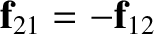, located at position vectors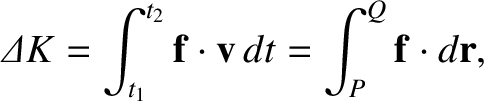and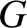, respectively. According to the preceding analysis, the acceleration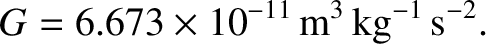of massas a result of the gravitational force exerted on it by masstakes the form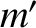(3.3)

Now, the-component of this acceleration is written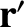(3.4)

where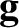and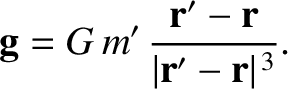. However, as is easily demonstrated,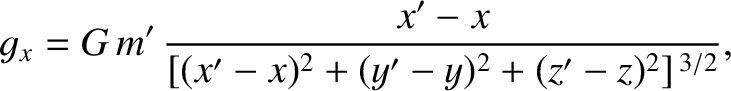(3.5)

Hence,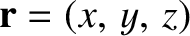(3.6)

with analogous expressions for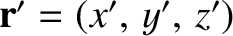and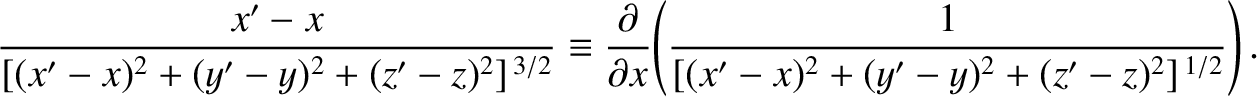. It follows that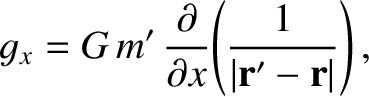(3.7)

where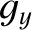(3.8)

is termed the gravitational potential. Of course, we can writein the form of Equation (3.7) only because gravity is a conservative force. (See Section 2.4.)

It is well known that gravity is a superposable force. In other words, the gravitational force exerted on some point mass by a collection of other point masses is simply the vector sum of the forces exerted on the former mass by each of the latter masses taken in isolation. It follows that the gravitational potential generated by a collection of point masses at a certain location in space is the sum of the potentials generated at that location by each point mass taken in isolation. Hence, using Equation (3.8), if there are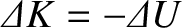point masses,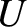(for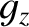), located at position vectors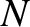, then the gravitational potential generated at position vectoris simply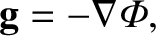(3.9)

Suppose, finally, that, instead of having a collection of point masses, we have a continuous mass distribution. In other words, let the mass at position vectorbe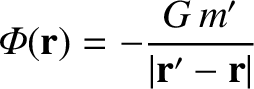, where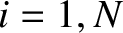is the local mass density, and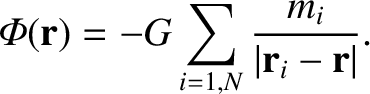a volume element. Summing over all space, and taking the limit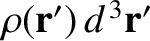, we find that Equation (3.9) yields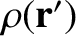(3.10)

where the integral is taken over all space. This is the general expression for the gravitational potential,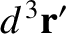, generated by a continuous mass distribution,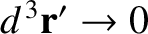.Next: Gravitational potential energy Up: Newtonian gravity Previous: Introduction
Richard Fitzpatrick 2016-03-31